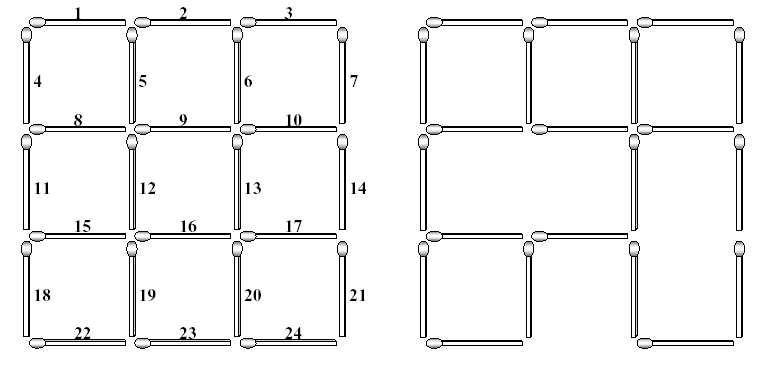### 题目链接：http://poj.org/problem?id=1084

Square Destroyer

Time Limit: 1000MS

Memory Limit: 10000K

Total Submissions: 3137

Accepted: 1323

Description

The left figure below shows a complete 3*3 grid made with 2*(3*4) (=24) matchsticks. The lengths of all matchsticks are one. You can find many squares of different sizes in the grid. The size of a square is the length of its side. In the grid shown in the left figure, there are 9 squares of size one, 4 squares of size two, and 1 square of size three. Each matchstick of the complete grid is identified with a unique number which is assigned from left to right and from top to bottom as shown in the left figure. If you take some matchsticks out from the complete grid, then some squares in the grid will be destroyed, which results in an incomplete 3*3 grid. The right figure illustrates an incomplete 3*3 grid after removing three matchsticks numbered with 12, 17 and 23. This removal destroys 5 squares of size one, 3 squares of size two, and 1 square of size three. Consequently, the incomplete grid does not have squares of size three, but still has 4 squares of size one and 1 square of size two.As input, you are given a (complete or incomplete) n*n grid made with no more than 2n(n+1) matchsticks for a natural number 5 <= n . Your task is to compute the minimum number of matchsticks taken out to destroy all the squares existing in the input n*n grid.

Input

The input consists of T test cases. The number of test cases (T ) is given in the first line of the input file. Each test case consists of two lines: The first line contains a natural number n , not greater than 5, which implies you are given a (complete or incomplete) n*n grid as input, and the second line begins with a nonnegative integer k , the number of matchsticks that are missing from the complete n*n grid, followed by k numbers specifying the matchsticks. Note that if k is equal to zero, then the input grid is a complete n*n grid; otherwise, the input grid is an incomplete n*n grid such that the specified k matchsticks are missing from the complete n*n grid.

Output

Print exactly one line for each test case. The line should contain the minimum number of matchsticks that have to be taken out to destroy all the squares in the input grid.

Sample Input

2
2
0
3
3 12 17 23

Sample Output

3
3

Source

Taejon 2001

#### 很明显的的重复覆盖模板题，主要是建图过程。

/* ***
Author :kuangbin
Created Time :2014/5/28 23:38:33
File Name :E:\2014ACM\专题学习\DLX\POJ1084.cpp
************************************************ */

#include <stdio.h>

#include <string.h>

#include

#include

#include

#include

#include

#include

#include

#include <math.h>

#include <stdlib.h>

#include <time.h>
using namespace std;

const int MaxM = 200;
const int MaxN = 100;
const int maxnode = 20000;
const int INF = 0x3f3f3f3f;
struct DLX
{
int n,m,size;
int U[maxnode],D[maxnode],R[maxnode],L[maxnode],Row[maxnode],Col[maxnode];
int H[MaxN],S[MaxM];
int ansd;
void init(int _n,int _m)
{
n = _n;
m = _m;
for(int i = 0;i <= m;i++)
{
S[i] = 0;
U[i] = D[i] = i;
L[i] = i-1;
R[i] = i+1;
}
R[m] = 0; L = m;
size = m;
for(int i = 1;i <= n;i++)H[i] = -1;
}
{
++S[Col[++size]=c];
Row[size] = r;
D[size] = D[c];
U[D[c]] = size;
U[size] = c;
D[c] = size;
if(H[r] < 0)H[r] = L[size] = R[size] = size;
else
{
R[size] = R[H[r]];
L[R[H[r]]] = size;
L[size] = H[r];
R[H[r]] = size;
}
}
void remove(int c)
{
for(int i = D[c];i != c;i = D[i])
L[R[i]] = L[i], R[L[i]] = R[i];
}
void resume(int c)
{
for(int i = U[c];i != c;i = U[i])
L[R[i]] = R[L[i]] = i;
}
bool v[MaxM];
int f()
{
int ret = 0;
for(int c = R;c != 0;c = R[c])v[c] = true;
for(int c = R;c != 0;c = R[c])
if(v[c])
{
ret++;
v[c] = false;
for(int i = D[c];i != c;i = D[i])
for(int j = R[i];j != i;j = R[j])
v[Col[j]] = false;
}
return ret;
}
void Dance(int d)
{
if(d + f() >= ansd)return;
if(R == 0)
{
if(d < ansd)ansd = d;
return;
}
int c = R;
for(int i = R;i != 0;i = R[i])
if(S[i] < S[c])
c = i;
for(int i = D[c];i != c;i = D[i])
{
remove(i);
for(int j = R[i];j != i;j = R[j])remove(j);
Dance(d+1);
for(int j = L[i];j != i;j = L[j])resume(j);
resume(i);
}
}
};
DLX g;

int id;
bool f;
int a;
int b;

int main()
{
//freopen(“in.txt”,”r”,stdin);
//freopen(“out.txt”,”w”,stdout);
int T;
int n;
scanf(“%d”,&T);
while(T–)
{
scanf(“%d”,&n);
int tot = (2*n+1)*n + n;
for(int i = 1;i <= tot;i++)f[i] = true;
int m,v;
scanf(“%d”,&m);
while(m–)
{
scanf(“%d”,&v);
f[v] = false;
}
int num = 0;
for(int i = 1;i <= n+1;i++)
{
for(int j = 1;j <= n;j++)
id[2i-1][j] = (++num);
if(i <= n)
{
for(int j = 0;j <= n;j++)
id[2
i][j] = (++num);
}
}
int cnt = 0;
for(int i = 1;i <= tot;i++)
if(f[i])
b[i] = (++cnt);
num = 0;
memset(a,-1,sizeof(a));
for(int i = 1;i <= n;i++)
for(int j = 1;j <= n;j++)
for(int k = 1;i+k-1 <= n && j+k-1 <= n;k++)
{
bool flag = true;
for(int x = 0;x < k;x++)
if(!f[id[2i-1][j+x]])
flag = false;
for(int x = 0;x < k;x++)
if(!f[id[2
(i+k-1)+1][j+x]])
flag = false;
for(int x = 0;x < k;x++)
if(!f[id[2(i+x)][j-1]])
flag = false;
for(int x = 0;x < k;x++)
if(!f[id[2
(i+x)][j+k-1]])
flag = false;
if(!flag)continue;
a[i][j][k] = (++num);
}
g.init(cnt,num);
for(int i = 1;i <= n;i++)
for(int j = 1;j <= n;j++)
for(int k = 1;i+k-1 <= n && j+k-1 <= n;k++)
if(a[i][j][k] != -1)
{
for(int x = 0;x < k;x++)
{
(i+k-1)+1][j+x]],a[i][j][k]);
(i+x)][j+k-1]],a[i][j][k]);
}
}
g.ansd = INF;
g.Dance(0);
printf(“%d\n”,g.ansd);
}
return 0;
}

------ 本文结束------
• 本文作者： kuangbin
• 本文链接： 50.html
• 版权声明： 本博客所有文章除特别声明外，均采用 CC BY-NC-SA 4.0 许可协议。转载请注明出处！
0%